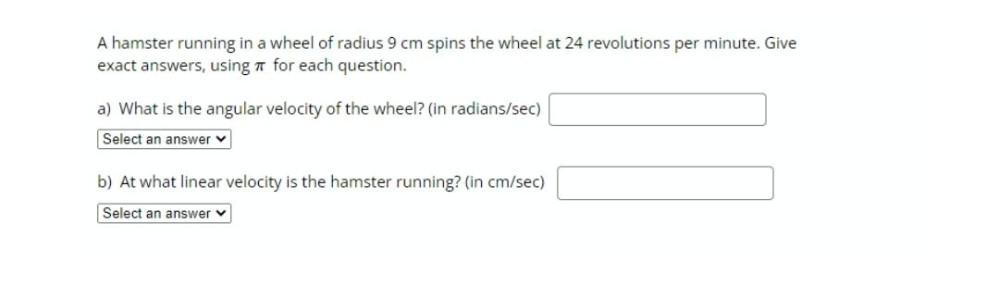Question:

# A hamster running in a wheel of radius 9 cm spins the wheel at 24 revolutions per minute. Give exact answers, using it for eachA hamster running in a wheel of radius 9 cm spins the wheel at 24 revolutions per minute. Give exact answers, using it for each question. a) What is the angular velocity of the wheel? (in radians/sec) Select an answer b) At what linear velocity is the hamster running? (in cm/sec) Select an answer